# Plot Smolyak multi-indices¶

The goal of this example is to plot the multi-indices used in Smolyak’s quadrature.

For a given dimension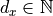and a given level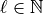Smolyak’s quadrature is the combination of tensorized univariate quadratures. These quadrature are defined by the set of multi-indices: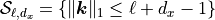where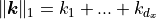is the 1-norm of the multi-index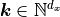.

The goal of this script is to plot the multi-indices involved in Smolyak’s quadrature for different values of the level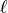in dimension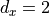.

import openturns as ot
import openturns.experimental as otexp
import openturns.viewer as otv
from matplotlib import pylab as plt


In the first example, we print the indices involved in Smolyak-Legendre quadrature of level 3. The multi-indices are computed using the computeCombination() method. Actually, the multi-indices do not actually depend on the underlying univariate quadratures, but this is required for the SmolyakExperiment class.

collection = [ot.GaussProductExperiment()] * 2
level = 3
print("level = ", level)
experiment = otexp.SmolyakExperiment(collection, level)
indices = experiment.computeCombination()
print(indices)

level =  3
[[2,1],[1,2],[3,1],[2,2],[1,3]]


We see that the multi-indices have a sum which is equal to either 3 or 4. In other words, these multi-indices belong to two different layers of constant 1-norms.

In order to see how this evolves depending on the level of the quadrature, the following function creates a 2D plot of the set of multi-indices.

def drawSmolyakIndices(level):
# Plot Smolyak indices of given level in 2 dimensions
collection = [ot.GaussProductExperiment()] * 2
experiment = otexp.SmolyakExperiment(collection, level)
indices = experiment.computeCombination()
sample = indices
graph = ot.Graph("L = %d" % (level), "k1", "k2", True)
cloud = ot.Cloud(sample)
cloud.setPointStyle("bullet")
return graph


In the following script, we create a grid of plots, where each graph corresponds to a given quadrature level. The bounding box of each graph is set to a constant value, so that all graphs have the same X and Y bounds.

levelMax = 8.0
boundingBox = ot.Interval([0.0] * 2, [levelMax] * 2)
nbrows = 2
nbcols = 3
grid = ot.GridLayout(nbrows, nbcols)
level = 1
for i in range(nbrows):
for j in range(nbcols):
graph = drawSmolyakIndices(level)
if i < nbrows - 1:
graph.setXTitle("")
if j > 0:
graph.setYTitle("")
graph.setBoundingBox(boundingBox)
grid.setGraph(i, j, graph)
level += 1
view = otv.View(grid, figure_kw={"figsize": (5.0, 3.0)})plt.show()